International
Tables for
Crystallography
Volume C
Mathematical, physical and chemical tables
Edited by E. Prince

International Tables for Crystallography (2006). Vol. C, ch. 8.4, pp. 703-704

## Section 8.4.2. The F distribution

E. Princea and C. H. Spiegelmanb

aNIST Center for Neutron Research, National Institute of Standards and Technology, Gaithersburg, MD 20899, USA, and bDepartment of Statistics, Texas A&M University, College Station, TX 77843, USA

### 8.4.2. The F distribution

| top | pdf |

Consider an unconstrained model with p parameters and a constrained one with q parameters, where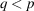. We wish to decide whether the constrained model represents an adequate fit to the data, or if the additional parameters in the unconstrained model provide, in some important sense, a better fit to the data. Provided the (pq) additional columns of the design matrix, A, are linearly independent of the previous q columns, the sum of squared residuals must be reduced by some finite amount by adjusting the additional parameters, but we must decide whether this improved fit would have occurred purely by chance, or whether it represents additional information.

Let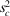and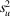be the weighted sums of squared residuals for the constrained and unconstrained models, respectively. If the constrained and unconstrained models are equally good representations of the data, and the weights have been assigned by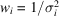, the expected values of the sums of squares are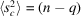and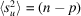, and, further, they should be distributed as χ2 with (nq) and (np) degrees of freedom, respectively. Also,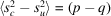, and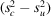is distributed as χ2 with (pq) degrees of freedom.andare not independent, butis the squared magnitude of a vector in a (pq)-dimensional subspace that is orthogonal to the (np)-dimensional space of. Therefore,andare independent, random variables, each with a χ2 distribution. Let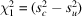,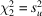, ν1 = pq, and ν2 = np. The ratio F =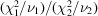should have a value close to one, even if the weights have relative rather than absolute values, but we need a measure of how far away from one this ratio can be before we must reject the hypothesis that the two models are equally good representations of the data. The conditional p.d.f. for F, given a value of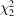, is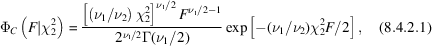and the marginal p.d.f. foris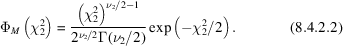The marginal p.d.f. for F is obtained by integration of the joint p.d.f.,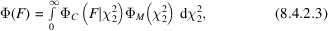yielding the result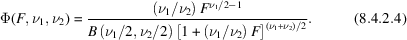This p.d.f. is known as the F distribution with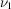and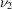degrees of freedom. Table 8.4.2.1gives the values of F for which the c.d.f.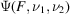is equal to 0.95 for various choices of ν1 and ν2. Fortran code for the program from which the table was generated appears in Prince (1994).

 Table 8.4.2.1| top | pdf | Values of the F ratio for which the c.d.f. ψ(F, ν1, ν2) has the value 0.95, for various choices of ν1 and ν21 2 4 8 15
10 4.9646 4.1028 3.4781 3.0717 2.8450
20 4.3512 3.4928 2.8661 2.4471 2.2033
30 4.1709 3.3158 2.6896 2.2662 2.0148
40 4.0847 3.2317 2.6060 2.1802 1.9245
50 4.0343 3.1826 2.5572 2.1299 1.8714
60 4.0012 3.1504 2.5252 2.0970 1.8364
80 3.9604 3.1108 2.4859 2.0564 1.7932
100 3.9361 3.0873 2.4626 2.0323 1.7675
120 3.9201 3.0718 2.4472 2.0164 1.7505
150 3.9042 3.0564 2.4320 2.0006 1.7335
200 3.8884 3.0411 2.4168 1.9849 1.7167
300 3.8726 3.0259 2.4017 1.9693 1.6998
400 3.8648 3.0183 2.3943 1.9616 1.6914
600 3.8570 3.0107 2.3868 1.9538 1.6831
1000 3.8508 3.0047 2.3808 1.9477 1.6764

The cumulative distribution functiongives the probability that the F ratio will be less than some value by chance if the models are equally consistent with the data. It is therefore a necessary, but not sufficient, condition for concluding that the unconstrained model gives a significantly better fit to the data thatbe greater than 1 − α, where α is the desired level of significance. For example, if= 0.95, the probability is only 0.05 that a value of F this large or greater would have been observed if the two models were equally good representations of the data.

Hamilton (1964) observed that the F ratio could be expressed in terms of the crystallographic weighted R index, which is defined, for refinement on |F| (and similarly for refinement on |F|2), by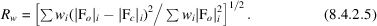Denoting byand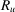the weighted R indices for the constrained and unconstrained models, respectively,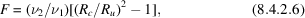and a c.d.f. for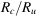can be readily derived from this relation. A significance test based onis known as Hamilton's R-ratio test; it is entirely equivalent to a test on the F ratio.

### References

Hamilton, W. C. (1964). Statistics in physical science: estimation, hypothesis testing and least squares. New York: Ronald Press.
Prince, E. (1994). Mathematical techniques in crystallography and materials science, 2nd ed. Berlin/Heidelberg/New York/London/Paris/Tokyo/Hong Kong/Barcelona/Budapest: Springer-Verlag.